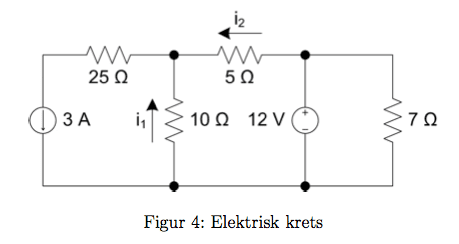# Need help with a really basic electrical circuit(KCL, KVL, Ohm's law)

• Engineering
Trippelganger

## Homework Statement

I should find i1 and i2.V = R*I
KCL
KVL

## The Attempt at a Solution

I have no idea where to even start, so I tried doing different things, but nothing that I do works. I am just really frustrated right now, for failing at doing such a simple thing. I would REALLY appreciate some good tips at how to think in this situation, and in what order I should set things up.

Mentor

## Homework Statement

I should find i1 and i2.V = R*I
KCL
KVL

## The Attempt at a Solution

I have no idea where to even start, so I tried doing different things, but nothing that I do works. I am just really frustrated right now, for failing at doing such a simple thing. I would REALLY appreciate some good tips at how to think in this situation, and in what order I should set things up.

Hi Trippleganger, Welcome to Physics Forums.

Have you attempted to apply KCL node analysis to the node where the three resistors meet? Can you show your attempt?

Trippelganger
Would that be V = R*I

i1 + i2 - 3A = 0
i1 + i2 = 3A

i1 = (12V/10 ohm)? (isn't the voltage the same for all elements in a parallel? I am so confused by this)
i2 = I don't even know where to start here

So as you can see, I'm pretty stuck. Giving me small hints so I can start to realize how to work is great! I absolutely don't want the whole solution, as that won't really help me in the long run! Just give me tips and how I should approach the problem and think about it! :)

Mentor
Would that be V = R*I

i1 + i2 - 3A = 0
i1 + i2 = 3A
That's good so far.
i1 = (12V/10 ohm)? (isn't the voltage the same for all elements in a parallel? I am so confused by this)
No, because the node voltage at the top of the 10 Ohm resistor is not 12V --- there's another resistor between that node and the 12V source.

The idea with nodal analysis is to solve for the individual node voltages. To proceed, you first assume that the node in question has some value of potential represented by a variable that you assign. Let's call the one for the node in question V1. The node to the right of the 5 Ohm resistor has its potential fixed by the 12V source, so no need to assign a variable there.

Given V1 at the node in question and 12V at the other node, can you write an expression for the current i2? Think Ohm's Law. Similarly, if the potential V1 is impressed across the 10 Ohm resistor, what's i1? Replace the unknowns i1 and i2 in your KCL equation above with these expressions and solve for V1. Once you have an actual value for V1 in hand you can go back and solve for i1 and i2.

Trippelganger
I'm really grateful for you helping me!

Is this the right thing to do?

V1 = 5 ohm * i2
V1 = 10 ohm * i1

V1/5 ohm + V1/10 ohm = 3A
V1 = 10V?

I don't get the right answer for that, so something is wrong :P

Mentor
No. Don't try to calculate V1 yet, use it to find expressions for i1 and i2. Use Ohm's law with the potential difference across the components. For example, i1 = (0V - V1)/10Ω.

Trippelganger
OK, so then it is:
(0V-V1)/10 ohm + (12V-V1)/5 ohm?

Thanks for being so patient! :)

Mentor
OK, so then it is:
(0V-V1)/10 ohm + (12V-V1)/5 ohm?

Yes, you're getting there. Now place that in context of your your KCL equation from before; The node has another current coming out of it, and together they all sum to zero, making a single equation. Solve for V1.

Trippelganger
Thanks a LOT! That did it for me! Hopefully, my brain is starting to learn to think in this way, and I won't have to bother you anymore :P But I will probably be back:P